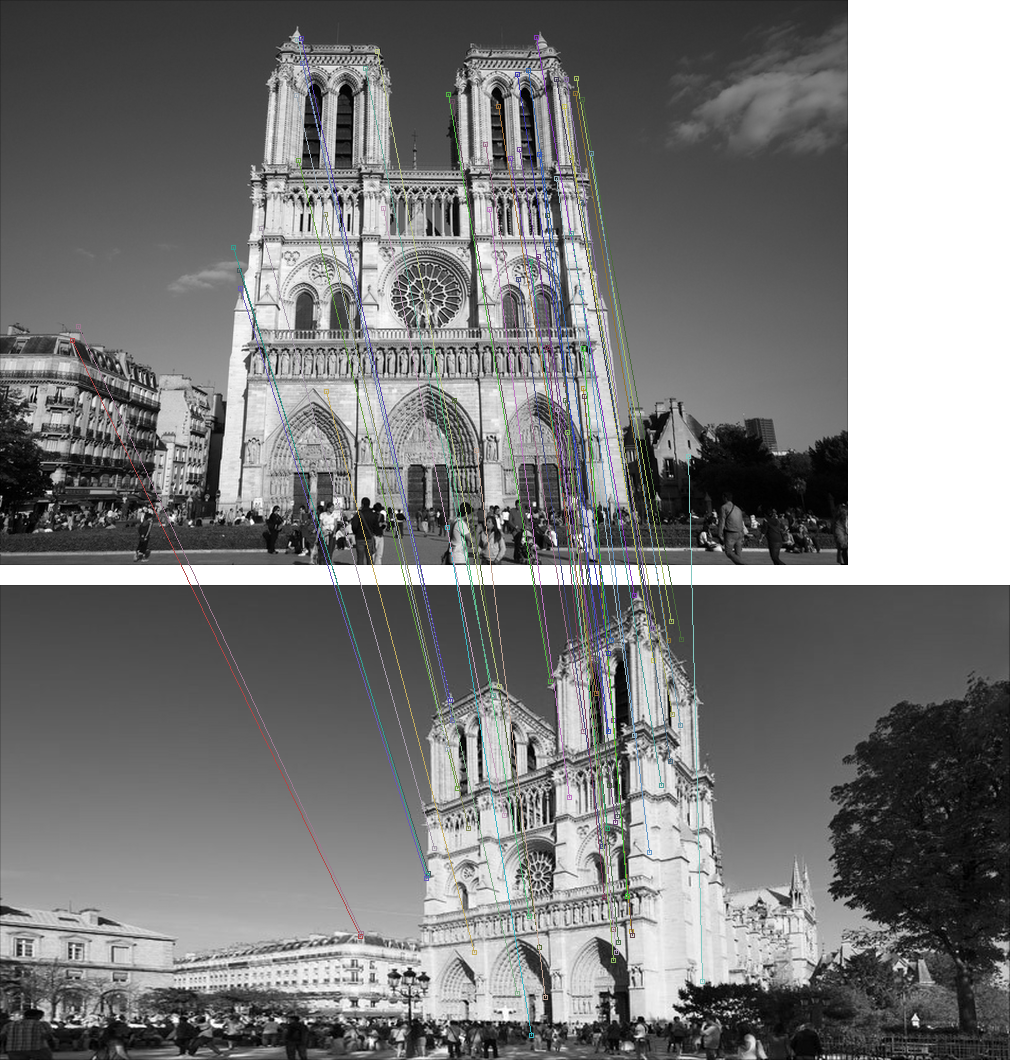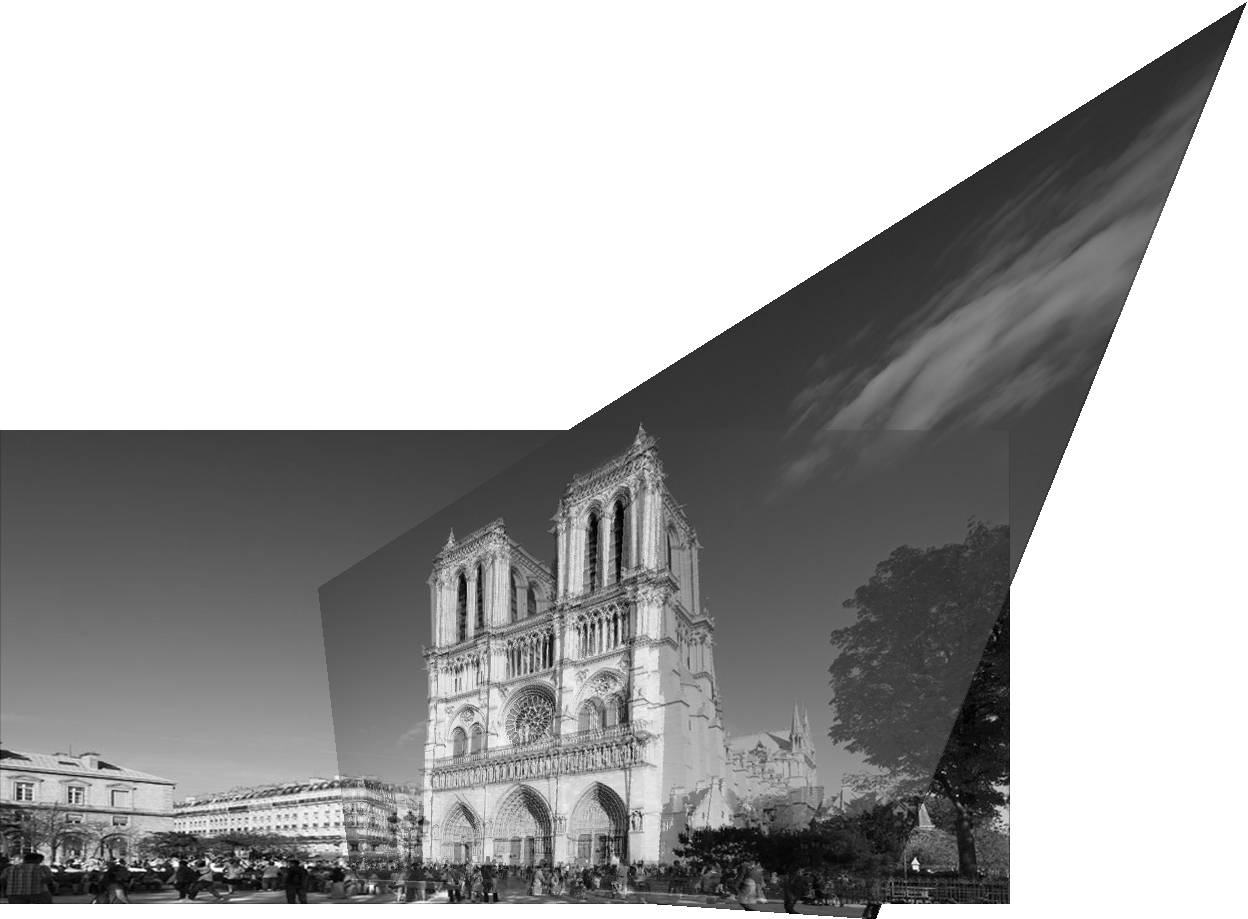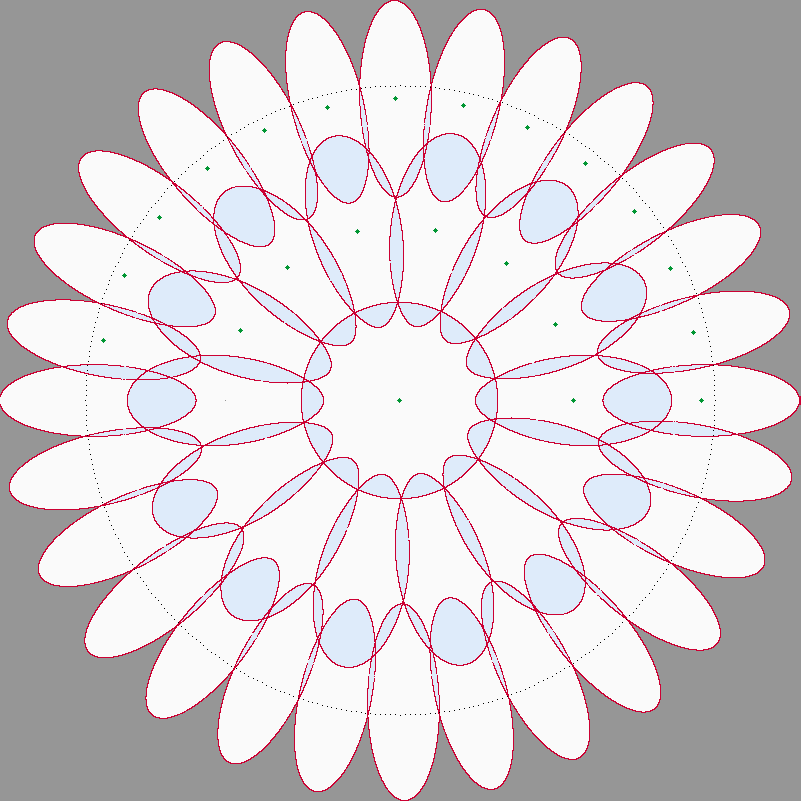# Fast Affine Invariant Image Matching

Full Title: “Fast Affine Invariant Image Matching”

Authors: Mariano Rodríguez, Julie Delon and Jean-Michel Morel

Journal: IPOL

Abstract: Methods performing Image Matching by Affine Simulation (IMAS) attain affine invariance by applying a finite set of affine transforms to the images before comparing them with a Scale Invariant Image Matching (SIIM) method like SIFT or SURF. We describe here how to optimize IMAS methods. First, we detail an algorithm computing a minimal discrete set of affine transforms to be applied to each image before comparison. It yields a full practical affine invariance at the lowest computational cost. The matching complexity of current IMAS algorithms is divided by about 4. Our approach also associates to each image an affine invariant set of descriptors, which is twice smaller than the set of descriptors usually used in IMAS methods, and only 6.4 times larger than the set of similarity invariant descriptors of SIIM methods. In order to reduce the number of false matches, which are inherently more frequent in IMAS approaches than in SIIM, we introduce the notion of hyper-descriptor, which groups descriptors whose keypoints are spatially close. Finally, we also propose a matching criterion allowing each keypoint of the query image to be matched with several keypoints of the target image, in order to deal with situations where an object is repeated several times in the target image.

Test it online (on IPOL)
Source code (on Github)

The above source code is a standalone C++ code for IMAS (as in IPOL) implementing Fast-Affine-{SIFT, RootSIFT, Half-SIFT, HALF-RootSIFT, SURF}.

Remark: This source code comes with a doxygen documentation.

### Notredame

The following is an example of matching two images with a significant change in point of view. In this case, Affine-RootSIFT was applied on the query (top) and target (bottom) images, and resulting matches were filtered with by ORSA Homography.The following shows the program’s output for this example.

--> Using 32 threads out of 32 for executing Optimal-Affine-RootSIFT <--

Covering Info:
Selecting optimal log1.7-covering for tilts up to 6
( t_1 phi_1 ... t_n phi_n ) = ( 2.6175699234 0.3980320096 5.1816802025 0.1983139962 )
Area ratio = 7.144

IMAS-Detector with RootSIFT...
4487 hyper-descriptors from 13180 SIIM descriptors have been found in 25 simulated versions of image 1
stats: group_min = 1 , group_mean = 2.937, group_max = 18
3655 hyper-descriptors from 10366 SIIM descriptors have been found in 25 simulated versions of image 2
stats: group_min = 1 , group_mean = 2.836, group_max = 28
IMAS-Detector accomplished in 1.44 seconds.

IMAS-Matcher...
387 possible matches have been found.
IMAS-Matcher accomplished in 0.72 seconds.

Filters...
-> Applying ORSA filter (Homography)
Imposed precision <= 24
nfa=10.3993 inliers=6 precision=12.2644 (iter=12)
nfa=4.75748 inliers=10 precision=9.41421 (iter=21)
nfa=2.16053 inliers=16 precision=18.7833 (iter=482)
nfa=-5.7019 inliers=23 precision=20.0082 (iter=643,sample=45,72,110,193)
nfa=-6.71704 inliers=26 precision=23.4279 (iter=667,sample=23,179,254,247)
nfa=-9.00678 inliers=28 precision=23.6846 (iter=758,sample=306,71,45,187)
nfa=-16.0474 inliers=33 precision=23.2265 (iter=785,sample=23,324,257,363)
nfa=-25.143 inliers=39 precision=22.776 (iter=837,sample=324,23,260,182)
nfa=-32.8 inliers=44 precision=22.7168 (iter=955,sample=324,182,276,111)
nfa=-48.9085 inliers=52 precision=20.936 (iter=962,sample=106,337,92,270)
nfa=-50.2798 inliers=56 precision=23.6407 (iter=992,sample=186,285,350,36)
nfa=-51.4467 inliers=50 precision=18.0402 (iter=1235,sample=363,199,270,13)
nfa=-51.9596 inliers=55 precision=21.9383 (iter=1304,sample=13,192,212,36)
nfa=-53.297 inliers=58 precision=23.7787 (iter=1635,sample=260,101,173,155)
Before refinement: Average/max error: 11.1799/23.3425
After  refinement: Average/max error: 9.9503/22.3047
The two images match! 58 matchings are identified. log(nfa)=-53.30.
*************** Homography ***************
[ 0.0828366 0.23572 317.441;  -0.34383 0.752014 158.308;  -0.00081302 0.000503007 1 ]
******************************************
Number of filtered matches =  58.
Filters were applied in 0.15 seconds.

Final number of matches =  58.

Done.


Let’s now take a look at the panorama in order to visually measure how good was the estimated homography.### IMAS on MATLAB

To have access to IMAS on MATLAB we propose to use the MEX compiler. The original code of both C++ implementations of IMAS (standalone or opencv) is handled through the MATLAB function “perform_IMAS.m” in the following repository: imas_analytics.

Remark: Follow the instructions in this repo to properly set up the link between IMAS and MATLAB.

### Finding near optimal coverings

The C++ code for finding near optimal coverings based on radius (initial visibility) and the region to cover is available in the following repository: imas_analytics.

First, go to the folder “opt_covering” and then compile in linux with :

 mkdir -p build && cd build && cmake .. && make


This code doesn’t have any documentation available yet but there exist a pseudo-code in the article to which this page is associated. Executing the program is very simple, the following example in bash is clear enough:

 r=1.6           # radius of disks
region=4.5      # region to be covered
N=2             # number of groups of concentric disks

epsilon=1.025   # Parameter for discretising annulus.
# Used to check for covering conditions

./opt_covering $r$region $epsilon$N


The above bash commands would return all parameters determining a near optimal covering. The following image was auto-generated by the above execution of this program.The present IMAS version shares with ASIFT the idea of simulating affine distortions. Two main differences are observed:

• The set of affine simulations. ASIFT generates overlapping affine distortions whereas this IMAS version applies near optimal coverings as in the paper Covering the Space of Tilts.
• The structure. ASIFT applies the SIFT method among all possible combinations of simulated query and target images; whereas this IMAS version compares in a single stage both set of hyper-descriptors coming from the query and target images.

#### Reproducing our comparison to ASIFT

To reproduce our results concerning ASIFT you can download the following file: tests_newIMAS_vs_oldIMAS.zip. This file contains the ASIFT code modified only to activate/deactivate filters, to obtain a more verbose mode, to add more descriptors (i.e. RootSIFT, HALF-SIFT), to select different coverings, etc. In any case, this version of ASIFT is a more complete one than the one proposed in IPOL. Once extracted, execute the following bash scripts:

# Compile both IMAS versions automatically
# The old version contains the ASIFT implementation
./compile.sh

# Compares the ASIFT covering against the near optimal one
# This under the ASIFT structure (i.e. No hyper-descriptors, etc)
./execute_coverings.sh graf1.png graf2.png

# Compares the ancient ASIFT structure to IMAS with hyper-descriptors
./execute_HD.sh graf1.png graf2.png


[ View on LinkedIn ]       [ View on Github ]